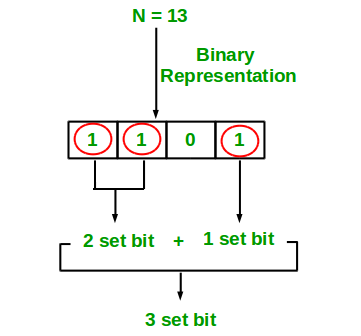# PHP Program to Count set bits in an integer

Write an efficient program to count number of 1s in binary representation of an integer.

Examples :

```Input : n = 6
Output : 2
Binary representation of 6 is 110 and has 2 set bits

Input : n = 13
Output : 3
Binary representation of 11 is 1101 and has 3 set bits
```## Recommended: Please solve it on “PRACTICE ” first, before moving on to the solution.

1. Simple Method Loop through all bits in an integer, check if a bit is set and if it is then increment the set bit count. See below program.

## PHP

 `>= 1; ` `    ``} ` `    ``return` `\$count``; ` `} ` ` `  `// Driver Code ` `\$i` `= 9; ` `echo` `countSetBits(``\$i``); ` ` `  `// This code is contributed by ajit ` `?> `

Output:

```2
```

Recursive Approach :

## PHP

 `> 1); ` `} ` ` `  `// Driver code ` ` `  `// get value from user ` `\$n` `= 9; ` ` `  `// function calling ` `echo` `countSetBits(``\$n``); ` ` `  `// This code is contributed by m_kit. ` `?> `

Output:

```2
```

Please refer complete article on Count set bits in an integer for more details!

My Personal Notes arrow_drop_up

Article Tags :

Be the First to upvote.

Please write to us at contribute@geeksforgeeks.org to report any issue with the above content.